GFG App
Open AppBrowser
Continue

# How to use hide() method in jQuery ?

The hide() method in jQuery is used for hiding the selected web elements. In this article, we will discuss the hide() method in detail. This method is generally used for effects or animation in jQuery. It also allows us to animate the behavior (transition) of hiding any specific element.

Syntax:

`.hide( duration, easing, complete )`

Parameters: This method has the following parameters:

• duration: It will determine how long any animation will run. It could be a String or Number. The possible values are “fast”, “slow”, or the time in milliseconds. The default value is 400 (in ms).
• easing: It will determine which easing function is being used for the transition of the element. The possible values are “swing” and “linear”. The default value is “swing”.
• complete: This function is called when the animation is completed. This function will be called each time per selected web element.

Note: If we don’t use any of the parameters, the element will just hide without any special transition or effect. We don’t have to use each parameter every time, we can use any one of these for our convenience.

Now let’s talk about how we can use this method to make things easy. We can use it to hide the selected element on a button click, on hover, and click on the element itself and we can also set a timer so that after the delay, the selected element will be hidden.

Let us see some examples of the hide() method for a better understanding of its working.

CDN link: The following jQuery library file is used in all the codes for its working which is included in the <head> section of each HTML code.

https://code.jquery.com/jquery-3.6.0.min.js

Example 1: In this example, we will set the hide() method to a button, so that when the button gets clicked, the selected element will be hidden. The elements could be an image, div, h1, etc.

## HTML

 `` `<``html` `lang``=``"en"``>` `<``head``>` `    ``` `    ``<``script` `src``=` `"https://code.jquery.com/jquery-3.6.0.min.js"` `            ``integrity``=` `"sha256-/xUj+3OJU5yExlq6GSYGSHk7tPXikynS7ogEvDej/m4="` `            ``crossorigin``=``"anonymous"``>` `        ```   `    ``<``style``>` `        ``* {` `            ``margin: 0px;` `            ``padding: 0px;` `            ``box-sizing: border-box;` `        ``}`   `        ``.container {` `            ``display: flex;` `            ``justify-content: center;` `            ``align-items: center;` `            ``flex-direction: column;` `            ``background-color: rgb(24, 24, 24);` `            ``height: 100vh;` `        ``}`   `        ``button {` `            ``margin: 50px;` `            ``height: 50px;` `            ``width: 100px;` `        ``}`   `        ``h1 {` `            ``color: green;` `        ``}` `    ``` ``   `<``body``>` `    ``<``div` `class``=``"container"``>` `        ``<``h1``><``b``>GeeksforGeeks`   `        ``<``button` `id``=``"btn"``>Hide` `    ```   `    ``` `    ``<``script``>` `        ``\$(document).ready(function () {` `            ``\$("#btn").click(function () {` `                ``\$("h1").hide();` `            ``});` `        ``});` `    ``` `` ``

Output: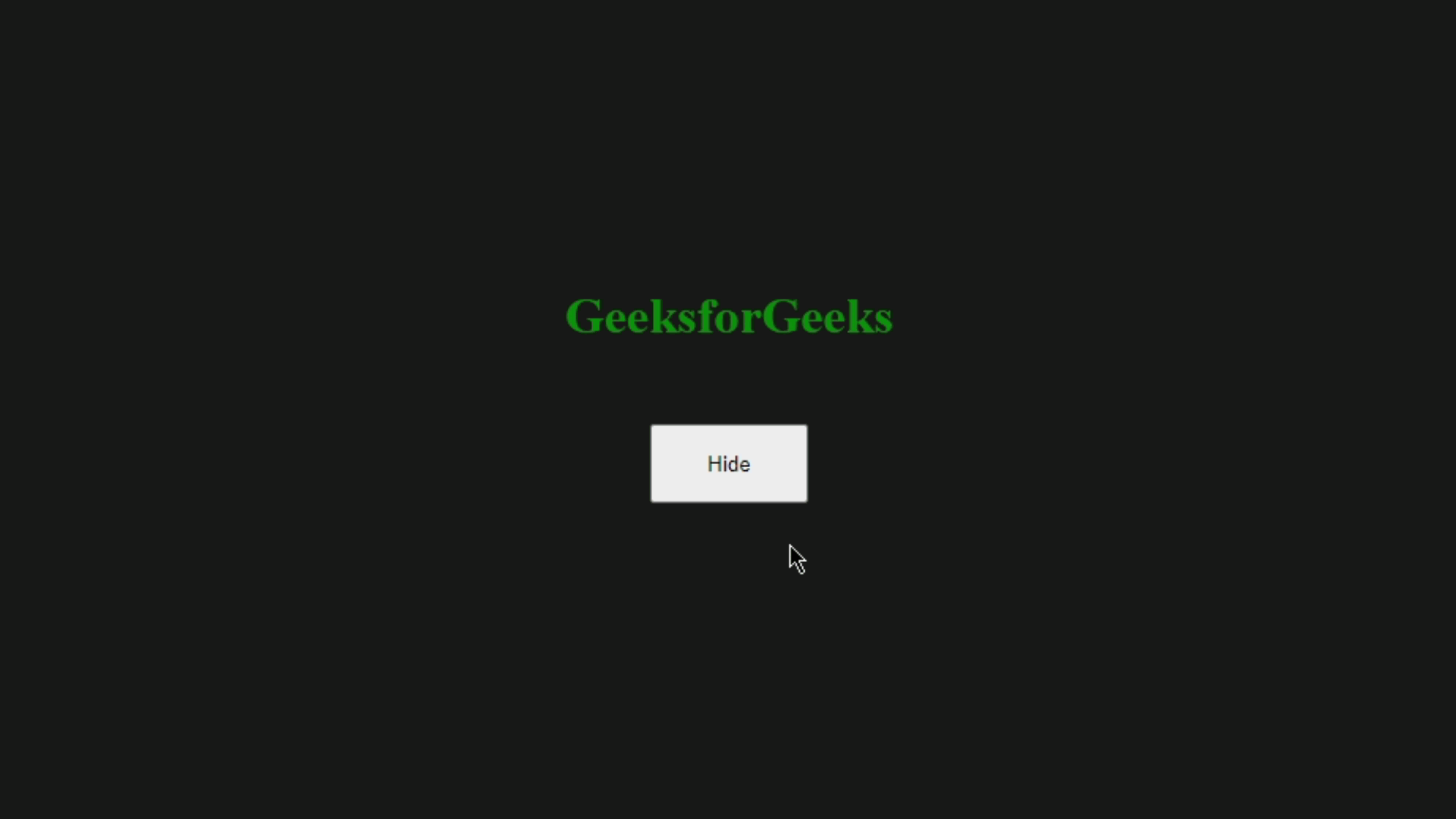Example 2: In the following example, if we hover over the selected element it will hide. Use this code snippet and replace the code in the <body> tag of Example 1 to get this result.

## HTML

 `` `<``html` `lang``=``"en"``>`   `<``head``>` `    ``` `    ``<``script` `src``=` `"https://code.jquery.com/jquery-3.6.0.min.js"` `            ``integrity``=` `"sha256-/xUj+3OJU5yExlq6GSYGSHk7tPXikynS7ogEvDej/m4="` `            ``crossorigin``=``"anonymous"``>` `        ``` `    ``<``style``>` `        ``* {` `            ``margin: 0px;` `            ``padding: 0px;` `            ``box-sizing: border-box;` `        ``}`   `        ``.container {` `            ``display: flex;` `            ``justify-content: center;` `            ``align-items: center;` `            ``flex-direction: column;` `            ``background-color: rgb(24, 24, 24);` `            ``height: 100vh;` `        ``}`   `        ``button {` `            ``margin: 50px;` `            ``height: 50px;` `            ``width: 100px;` `        ``}`   `        ``h1 {` `            ``color: green;` `        ``}` `    ``` ``   `<``body``>` `    ``<``div` `class``=``"container"``>` `        ``<``h1``><``b``>GeeksforGeeks` `    ```   `    ``` `    ``<``script``>` `        ``\$(document).ready(function () {` `            ``\$("h1").hover(function () {` `                ``\$("h1").hide();` `            ``});` `        ``});` `    ``` `` ``

Output: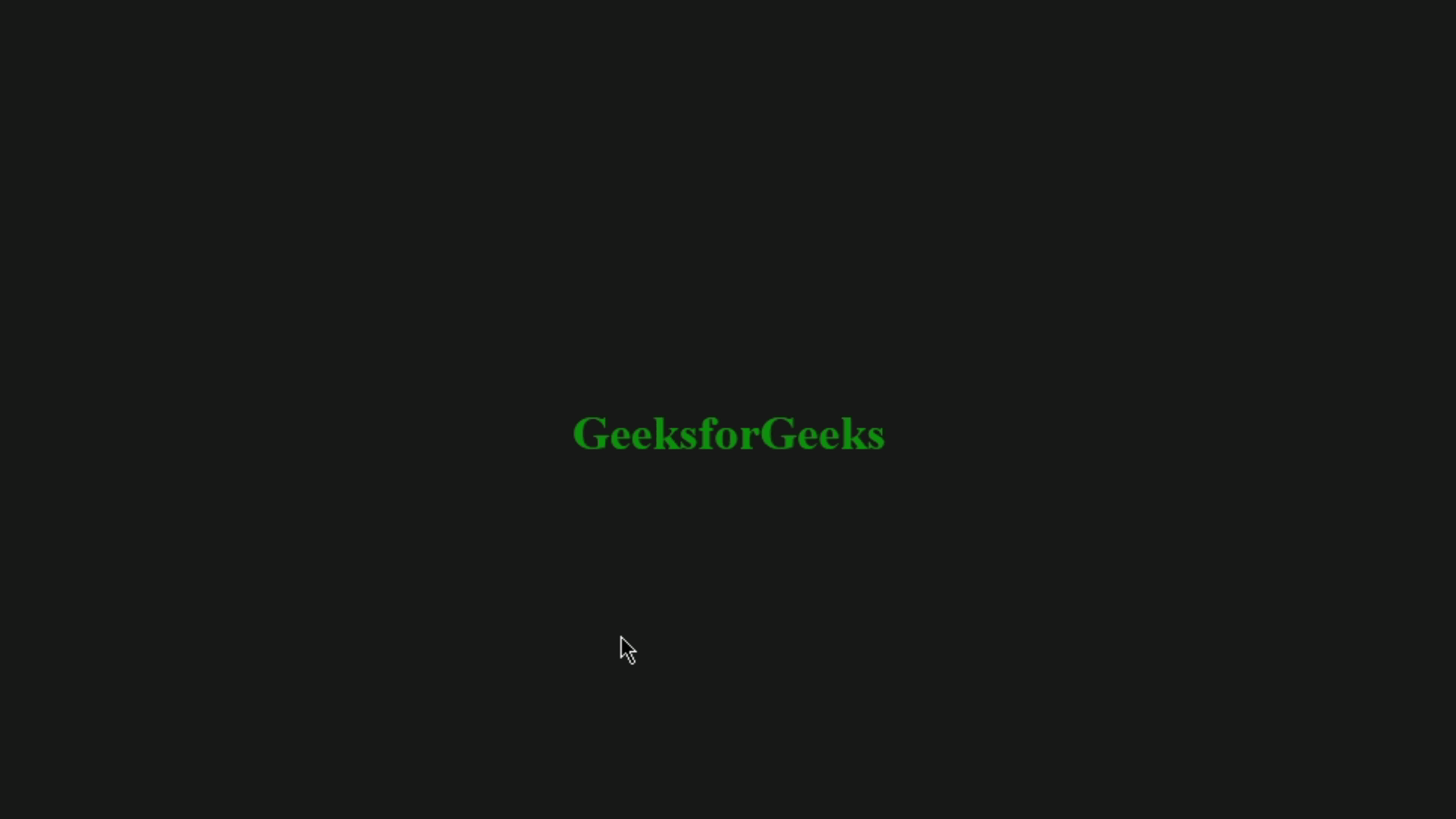Example 3: For this example, we will set a timer of 2 seconds, after passing that time the selected element will be hidden whenever we click the hide button. Use this code snippet and replace the code inside the <body> tag of example 1 to get this result.

## HTML

 `` `<``html` `lang``=``"en"``>`   `<``head``>` `    ``` `    ``<``script` `src``=` `"https://code.jquery.com/jquery-3.6.0.min.js"` `            ``integrity``=` `"sha256-/xUj+3OJU5yExlq6GSYGSHk7tPXikynS7ogEvDej/m4="` `            ``crossorigin``=``"anonymous"``>` `        ``` `    ``<``style``>` `        ``* {` `            ``margin: 0px;` `            ``padding: 0px;` `            ``box-sizing: border-box;` `        ``}`   `        ``.container {` `            ``display: flex;` `            ``justify-content: center;` `            ``align-items: center;` `            ``flex-direction: column;` `            ``background-color: rgb(24, 24, 24);` `            ``height: 100vh;` `        ``}`   `        ``button {` `            ``margin: 50px;` `            ``height: 50px;` `            ``width: 100px;` `        ``}`   `        ``h1 {` `            ``color: green;` `        ``}` `    ``` ``   `<``body``>` `    ``<``div` `class``=``"container"``>` `        ``<``h1``><``b``>GeeksforGeeks` `        ``<``button` `id``=``"btn"``>Hide` `    ```   `    ``` `    ``<``script``>` `        ``\$(document).ready(function () {` `            ``\$("#btn").click(function () {` `                ``\$("h1").delay(2000).hide("fast");` `            ``});` `        ``});` `    ``` `` ``

Output: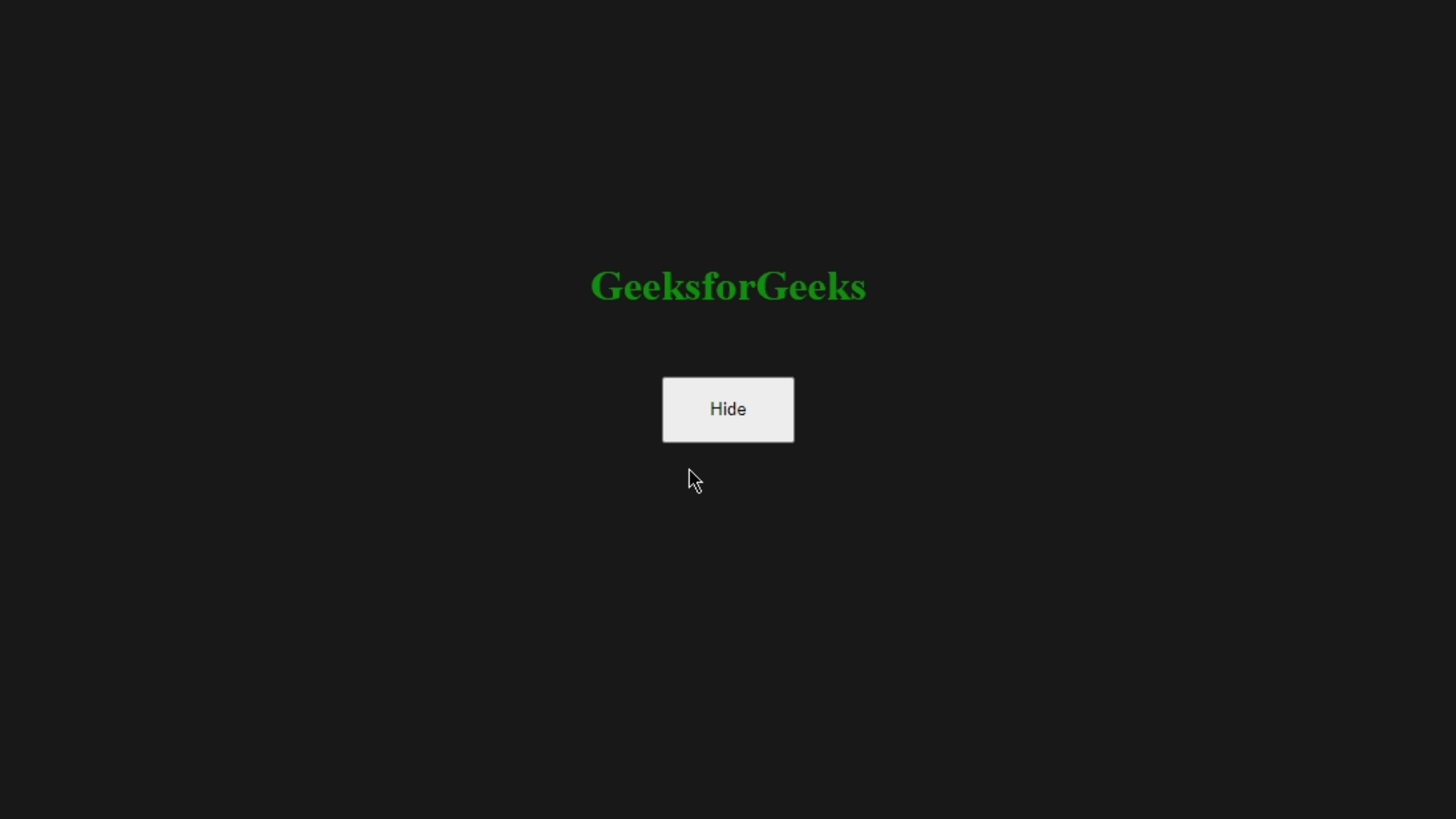Example 4: In this example, we will use the duration parameter and apply slow and fast hiding transitions of the selected element. We have two text elements and two buttons, one will hide the text slowly and the second one will hide the text instantly. Use this code snippet in Example 1.

## HTML

 `` `<``html` `lang``=``"en"``>`   `<``head``>` `    ``` `    ``<``script` `src``=` `"https://code.jquery.com/jquery-3.6.0.min.js"` `            ``integrity``=` `"sha256-/xUj+3OJU5yExlq6GSYGSHk7tPXikynS7ogEvDej/m4="` `            ``crossorigin``=``"anonymous"``>` `        ``` `    ``<``style``>` `        ``* {` `            ``margin: 0px;` `            ``padding: 0px;` `            ``box-sizing: border-box;` `        ``}`   `        ``.container {` `            ``display: flex;` `            ``justify-content: center;` `            ``align-items: center;` `            ``flex-direction: column;` `            ``background-color: rgb(24, 24, 24);` `            ``height: 100vh;` `        ``}`   `        ``button {` `            ``margin: 50px;` `            ``height: 50px;` `            ``width: 100px;` `        ``}`   `        ``h1 {` `            ``color: green;` `        ``}` `    ``` ``   `<``body``>` `    ``<``div` `class``=``"container"``>` `        ``<``h1``><``b``>GeeksforGeeks` `        ``<``button` `id``=``"btn"``>Slow hide` `        ``<``h2``><``b``>GeeksforGeeks` `        ``<``button` `id``=``"btn1"``>Fast hide` `    ```   `    ``` `    ``<``script``>` `        ``\$(document).ready(function () {` `            ``\$("#btn").click(function () {` `                ``\$("h1").hide("slow");` `            ``});` `            ``\$("#btn1").click(function () {` `                ``\$("h2").hide("fast");` `            ``});` `        ``}` `        ``);` `    ``` `` ``

Output: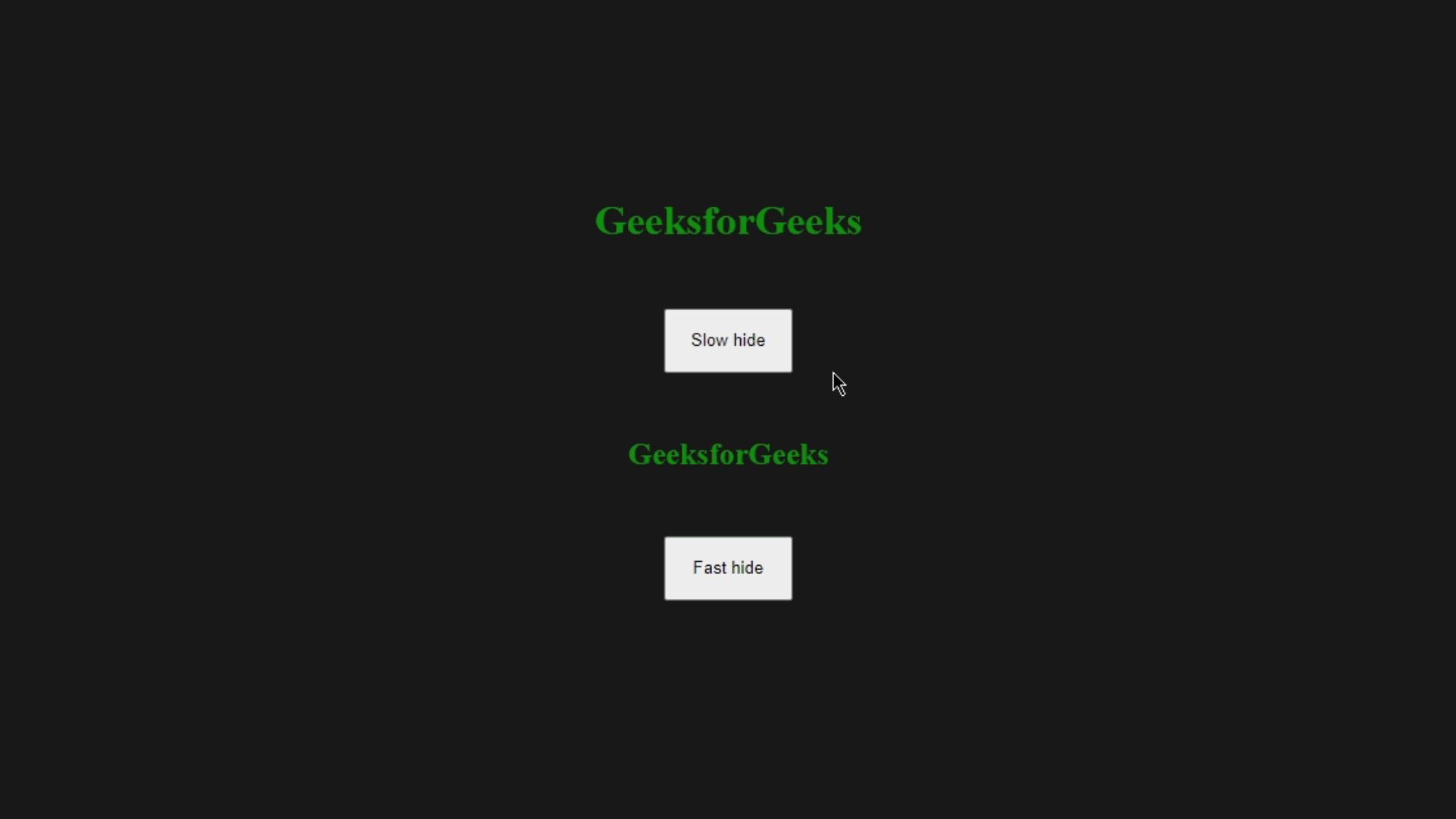Example 5: For this example, we will see how we can use the callback function so that when the hiding effect will complete, the function will be called. We will set an alert message after the completion of the hiding effect. Use this code snippet in Example 1.

## HTML

 `` `<``html` `lang``=``"en"``>`   `<``head``>` `    ``` `    ``<``script` `src``=` `"https://code.jquery.com/jquery-3.6.0.min.js"` `            ``integrity``=` `"sha256-/xUj+3OJU5yExlq6GSYGSHk7tPXikynS7ogEvDej/m4="` `            ``crossorigin``=``"anonymous"``>` `        ``` `    ``<``style``>` `        ``* {` `            ``margin: 0px;` `            ``padding: 0px;` `            ``box-sizing: border-box;` `        ``}`   `        ``.container {` `            ``display: flex;` `            ``justify-content: center;` `            ``align-items: center;` `            ``flex-direction: column;` `            ``background-color: rgb(24, 24, 24);` `            ``height: 100vh;` `        ``}`   `        ``button {` `            ``margin: 50px;` `            ``height: 50px;` `            ``width: 100px;` `        ``}`   `        ``h1 {` `            ``color: green;` `        ``}` `    ``` ``   `<``body``>` `    ``<``div` `class``=``"container"``>` `        ``<``h1``><``b``>GeeksforGeeks` `        ``<``button` `id``=``"btn"``>Slow hide` `    ```   `    ``` `    ``<``script``>` `        ``\$(document).ready(function () {` `            ``\$("#btn").click(function () {` `                ``\$("h1").hide("slow", function () {` `                    ``alert('The text is hidden!')` `                ``});` `            ``});` `        ``}` `        ``);` `    ``` `` ``

Output: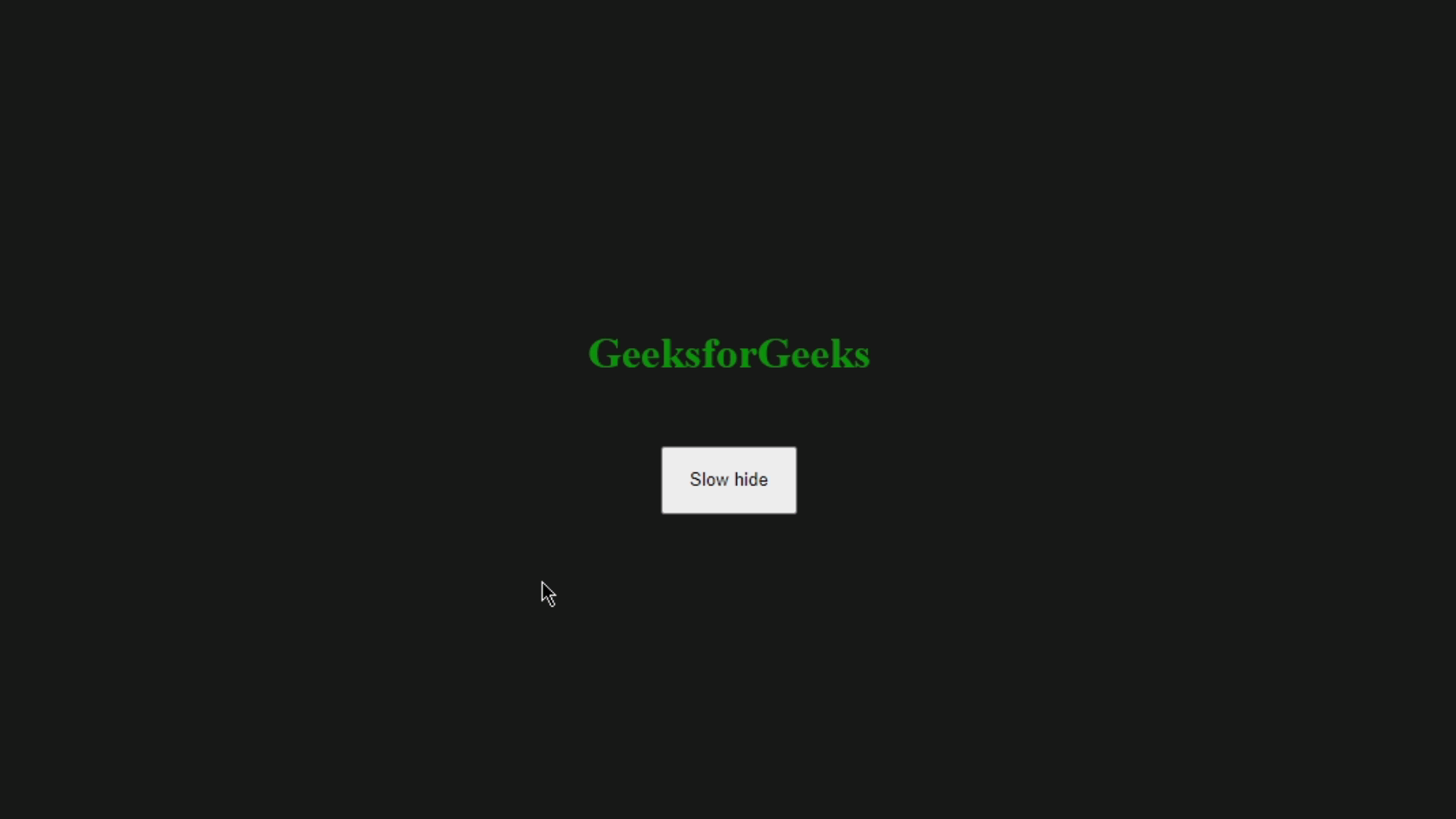My Personal Notes arrow_drop_up